# 第45回 | 解析并执行 shell 命令低并发编程 |30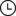2022-08-03 08:34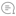0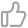00

https://github.com/sunym1993/flash-linux0.11-talk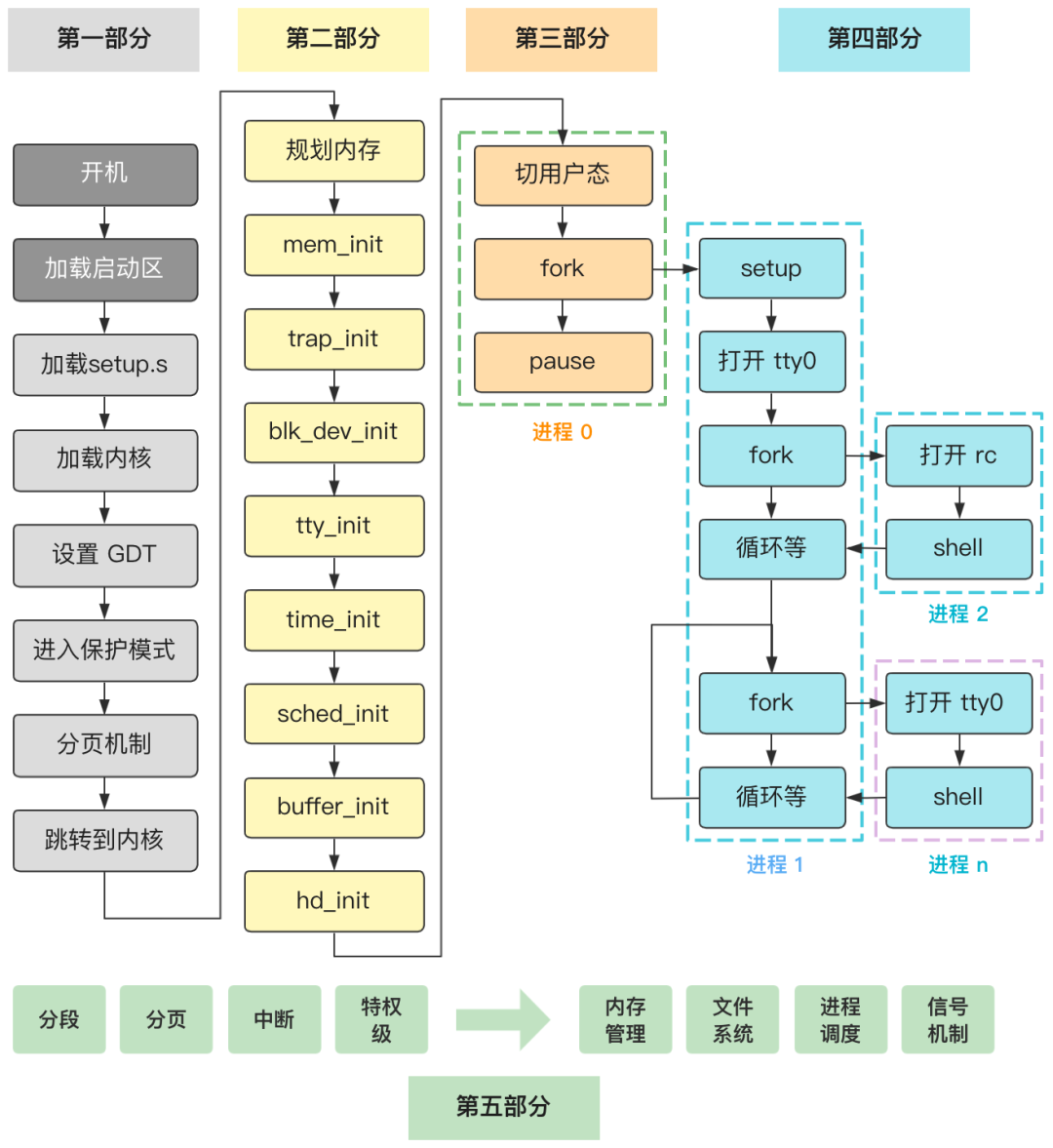------- 正文开始 -------

``name:flashage:28language:java``

``[root@linux0.11] cat info.txt | wc -l3````// xv6-public sh.cint main(void) {    static char buf;    // 读取命令    while(getcmd(buf, sizeof(buf)) >= 0){        // 创建新进程        if(fork() == 0)            // 执行命令            runcmd(parsecmd(buf));        // 等待进程退出        wait();    }}``

``// xv6-public sh.cvoid runcmd(struct cmd *cmd) {    ...    switch(cmd->type) {        ...        case EXEC:        ecmd = (struct execcmd*)cmd;        ...        exec(ecmd->argv, ecmd->argv);        ...         break;            case REDIR: ...        case LIST: ...        case PIPE: ...        case BACK: ...    }}``

``[root@linux0.11] cat info.txt | wc -l``

``// xv6-public sh.cvoid runcmd(struct cmd *cmd) {    ...    int p;    ...    case PIPE:        pcmd = (struct pipecmd*)cmd;        pipe(p);        if(fork() == 0) {            close(1);            dup(p);            close(p);            close(p);            runcmd(pcmd->left);        }        if(fork() == 0) {            close(0);            dup(p);            close(p);            close(p);            runcmd(pcmd->right);        }        close(p);        close(p);        wait(0);        wait(0);        break;    ...}``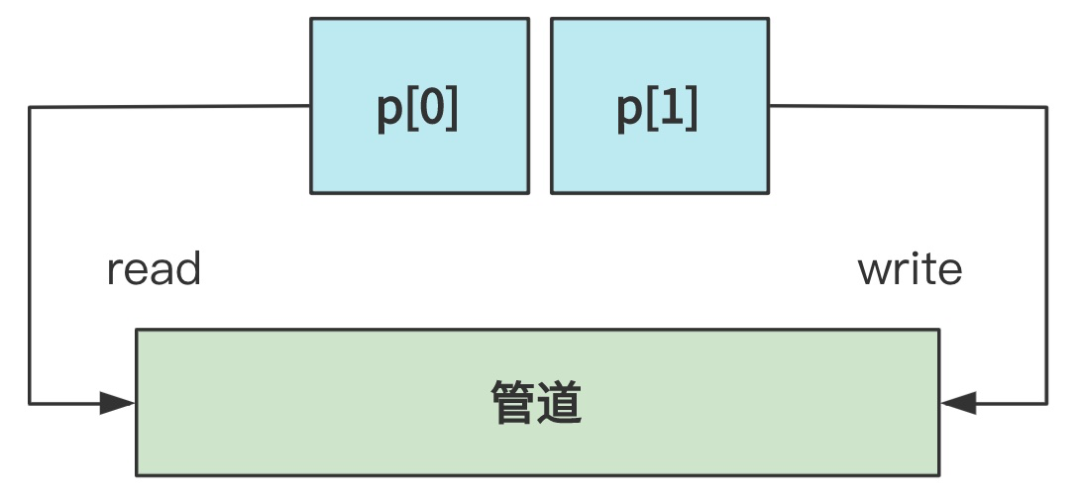``// xv6-public sh.cvoid runcmd(struct cmd *cmd) {    ...    int p;    ...    case PIPE:        pcmd = (struct pipecmd*)cmd;        pipe(p);        if(fork() == 0) {            close(1);            dup(p);            close(p);            close(p);            runcmd(pcmd->left);        }        if(fork() == 0) {            close(0);            dup(p);            close(p);            close(p);            runcmd(pcmd->right);        }        close(p);        close(p);        wait(0);        wait(0);        break;    ...}``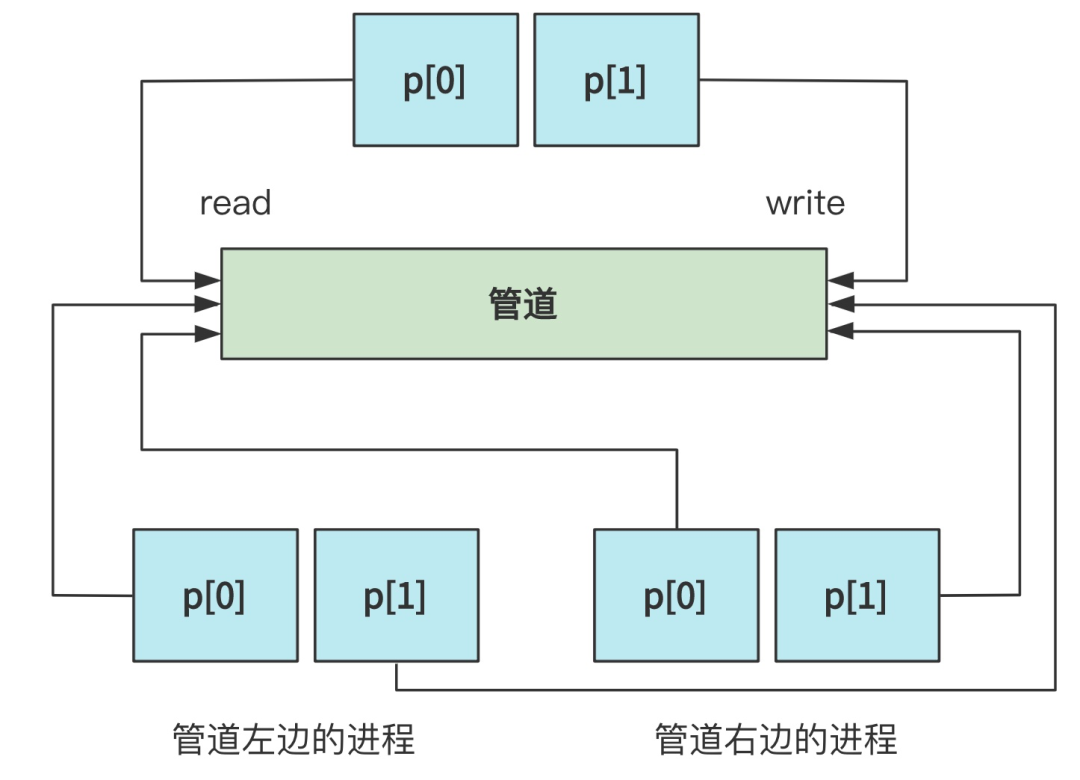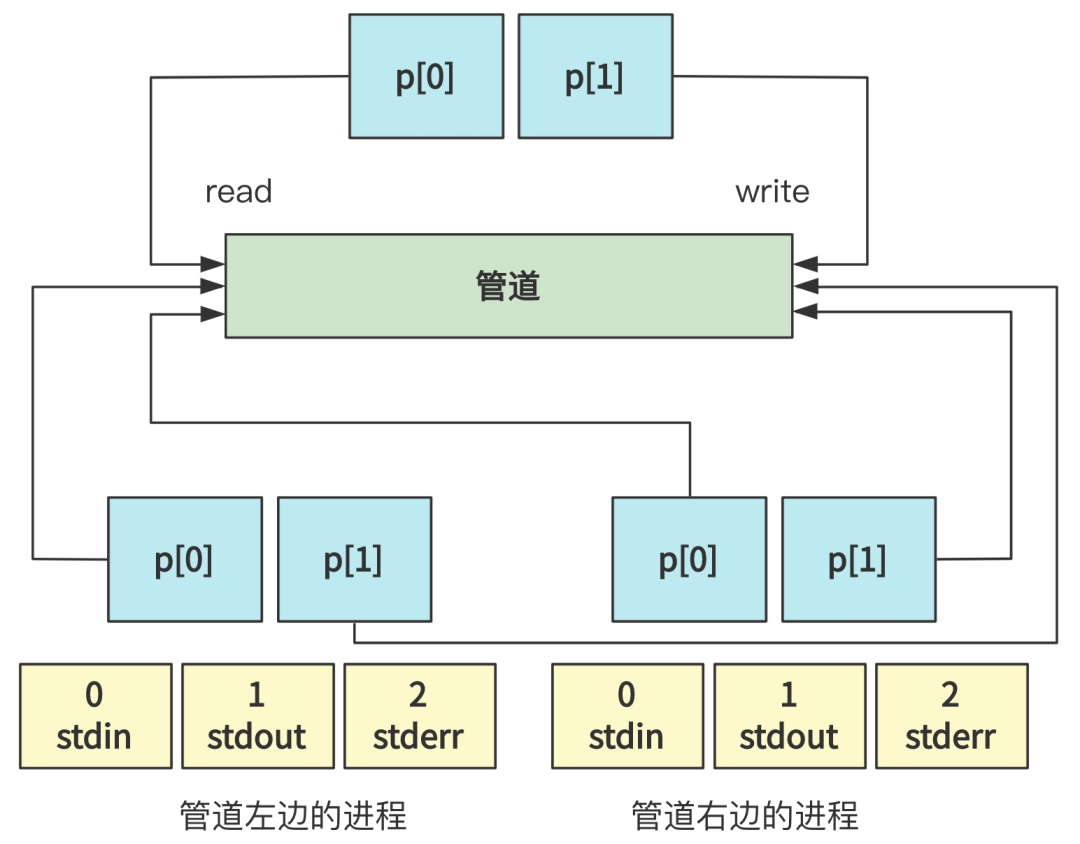``// fs/pipe.c...if(fork() == 0) {    close(1);    dup(p);    close(p);    close(p);    runcmd(pcmd->left);}...````// fs/pipe.c...if(fork() == 0) {    close(0);    dup(p);    close(p);    close(p);    runcmd(pcmd->right);}...``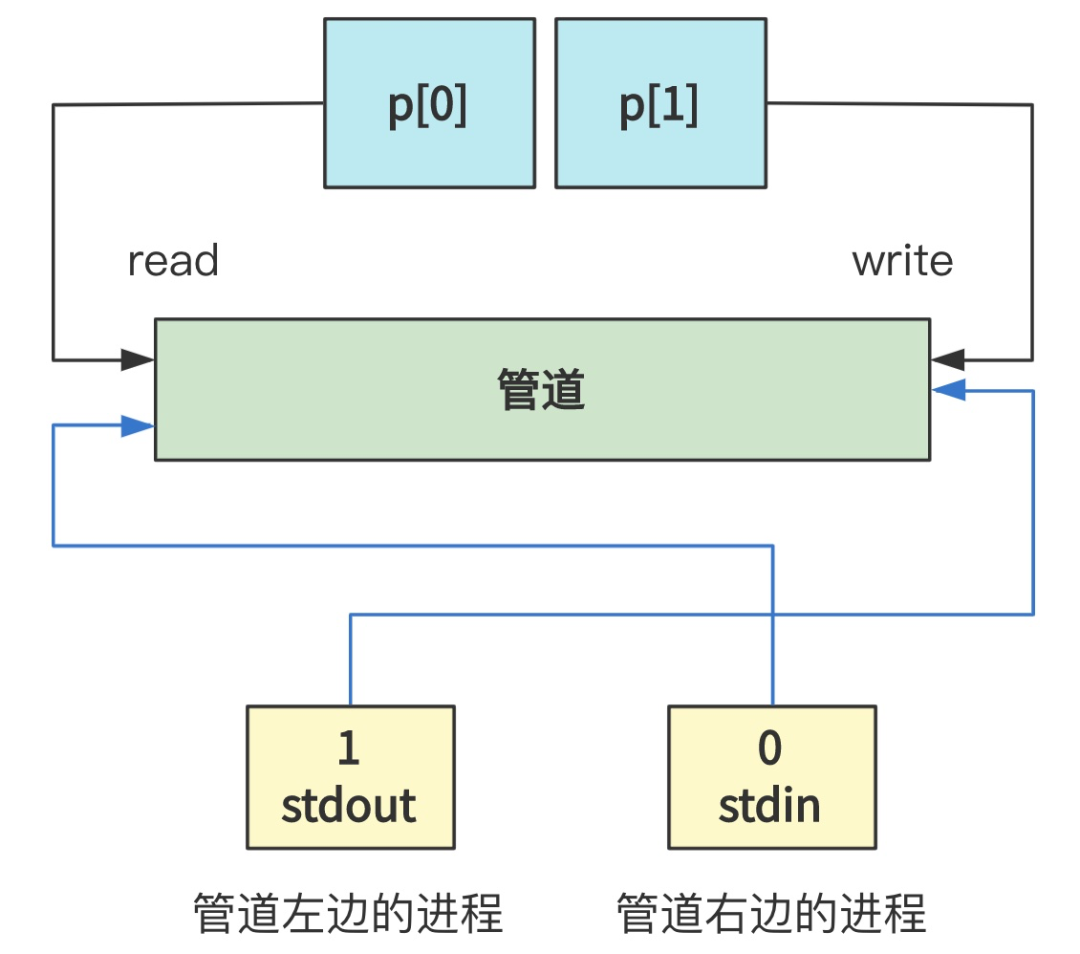``// xv6-public sh.cvoid runcmd(struct cmd *cmd) {    ...    pipe(p);    if(fork() == 0) {...}    if(fork() == 0) {...}    // 父进程    close(p);    close(p);    ...}``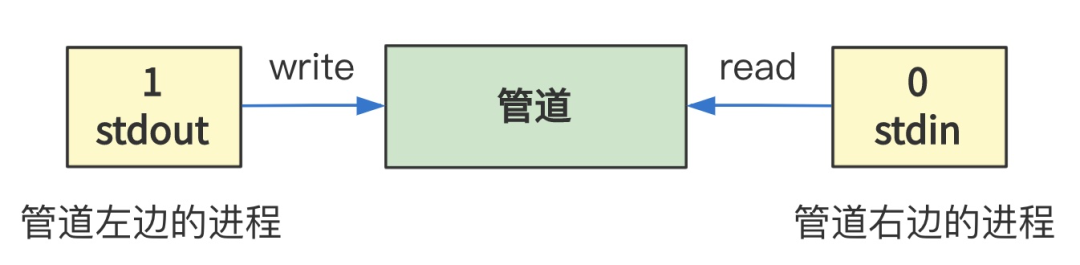``// fs/pipe.cint sys_pipe(unsigned long * fildes) {    struct m_inode * inode;    struct file * f;    int fd;    for(int i=0,j=0; j<2 && i<NR_FILE; i++)        if (!file_table[i].f_count)            (f[j++]=i+file_table)->f_count++;    ...    for(int i=0,j=0; j<2 && i<NR_OPEN; i++)        if (!current->filp[i]) {            current->filp[ fd[j]=i ] = f[j];            j++;        }    ...    if (!(inode=get_pipe_inode())) {        current->filp[fd] = current->filp[fd] = NULL;        f->f_count = f->f_count = 0;        return -1;    }    f->f_inode = f->f_inode = inode;    f->f_pos = f->f_pos = 0;    f->f_mode = 1;       /* read */    f->f_mode = 2;       /* write */    put_fs_long(fd,0+fildes);    put_fs_long(fd,1+fildes);    return 0;}``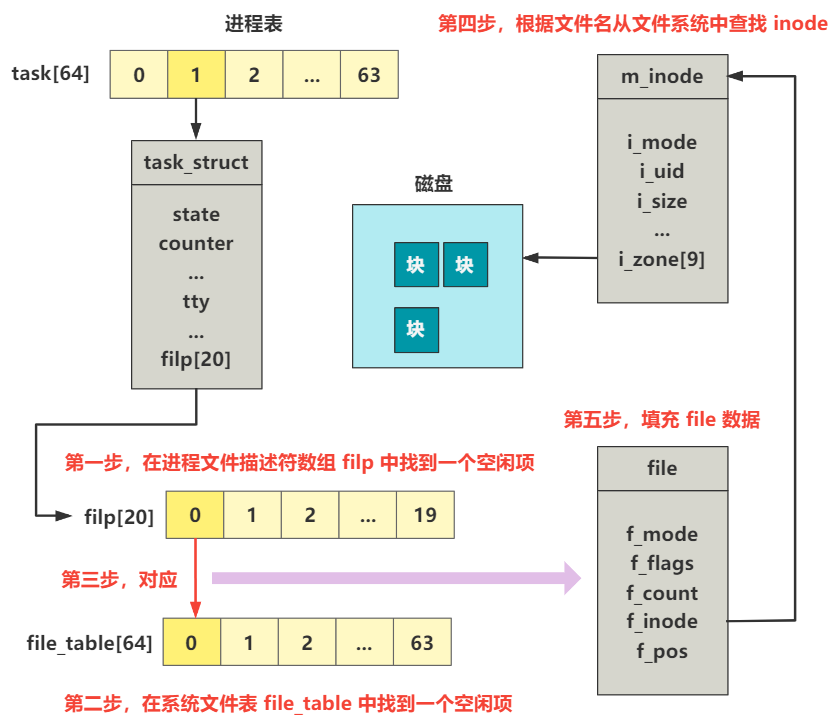``// fs.h#define PIPE_HEAD(inode) ((inode).i_zone)#define PIPE_TAIL(inode) ((inode).i_zone)// inode.cstruct m_inode * get_pipe_inode(void) {    struct m_inode *inode = get_empty_inode()；    inode->i_size=get_free_page()；    inode->i_count = 2; /* sum of readers/writers */    PIPE_HEAD(*inode) = PIPE_TAIL(*inode) = 0;    inode->i_pipe = 1;    return inode;}``

OK，管道的原理在这里就说完了，最终我们就是实现了一个进程的输出指向了另一个进程的输入。``// xv6-public sh.cvoid runcmd(struct cmd *cmd) {    ...    switch(cmd->type) {        ...        case EXEC:        ecmd = (struct execcmd*)cmd;        ...        exec(ecmd->argv, ecmd->argv);        ...         break;            case REDIR: ...        case LIST: ...        case PIPE: ...        case BACK: ...    }}``

EXEC 类型会执行到 exec 这个方法，在 Linux 0.11 中，最终会通过系统调用执行到 sys_execve 方法。

------- 关于本系列 -------00

暂无评论~~
Ctrl+Enter